# New SAT Math - Calculator : Triangle Similarity

## Example Questions

### Example Question #1 : Similar Triangles

What does the scale factor of a dilation need to be to ensure that triangles are not only similar but also congruent?Explanation:

The scale factor of a dilation tells us what we multiply corresponding sides by to get the new side lengths. In this case, we want these lengths to be the same to get congruent triangles. Thus, we must be looking for the multiplicative identity, which is 1.

### Example Question #1 : Identifying Similar Triangles

Which of the following is not a theorem to prove that triangles are similar?

SAS

SSS

ASA

AA

HL

ASA

Explanation:

ASA (Angle Side Angle) is a theorem to prove triangle congruency.

In this case, we only need two angles to prove that two triangles are similar, so the last side in ASA is unnecessary for this question.

For this purpose, we use the theorem AA instead.

### Example Question #3 : Similar Triangles

One triangle has side measures,, and. Another has side lengths,, and. Are these triangles similar?

There is not enough information given.

Yes

No

Those can't be the side lengths of triangles.

Triangles can't be similar!

No

Explanation:

Two triangles are similar if and only if their side lengths are proportional.

In this case, two of the sides are proportional, leading us to a scale factor of 2.

However, with the last side,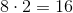which is not our side length.

Thus, these pair of sides are not proportional and therefore our triangles cannot be similar.

### Example Question #2 : Triangle Similarity

Which of the following triangles are similar?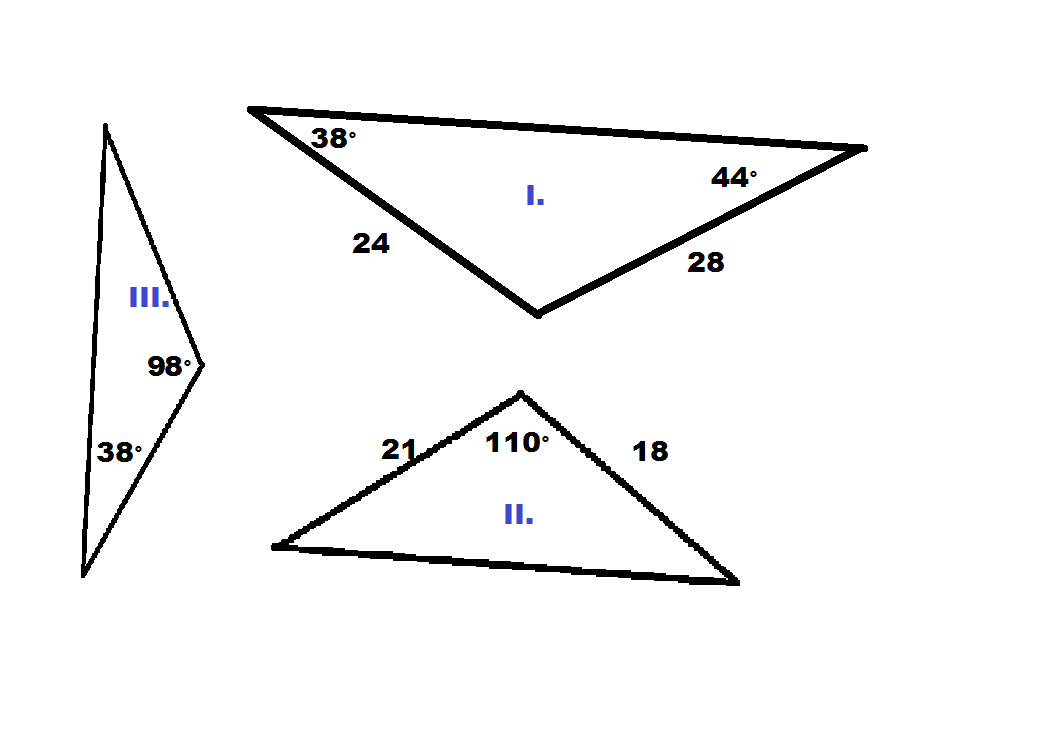II and III

None of the triangles are similar

I and II

I and III

I, II, and III

I and III

Explanation:

We must remember that there are three ways to prove triangles are similar.

1. At least two angles in one triangle are congruent to angles in another (AA)
2. All three pairs of corresponding sides are proportional (SSS)
3. Two pairs of corresponding sides are proportional and the angles between those sides are congruent (SAS)

Comparing triangles I and II, we only have one angle and two sides in trinagle II, so attempting to use either AA or SSS for similarity will not work, leaving SAS as the only option.  If we compare the two given sides in each triangle, we notice that the ratio of the longer side in triangle I to the longer side in triangle II is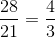The ratio of the shorter sides in each triangle are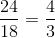Notice we have equal ratios and thus a proportion.  However, we still must confirm that the included angles are congruent.  The measure for this angle is not given in triangle I, but we can calculate since all three angles must add up to 180 degrees.  Calculation tells us that the measure is 98 degrees, which unfortunately does not equal the 110 from triangle II.  Therefore, we have no SAS and therefore no similarity between I and II.

Transitioning to I and III, we only have angles in triangle III, so we are unable to use either SSS or SAS.  However, we previously calculated the measure third angle in triangle I to be 98.  Therefore, two of our angles are congruent, meaning we have AA and thus similarity.

Regarding II and III, we can use some logic.  Since we know I and III are similar, then if II and III were also similar, then we could use the transitive property to conclude that I and II are also similar.  But we know this is false, so II and III cannot be similar.

Therefore, the only two similar triangles are I and III.

### Example Question #3 : Triangle Similarity

One triangle has side measures 2, 4, and 5. Another has sides 4, 8, and 10. Are these triangles similar?

No

There is not enough information

They are congruent triangles

Yes

Yes

Explanation:

To determine if the triangles are similar, set up a proportion.

2/4 = 4/8 = 5/10

When we do this, we cross multiply to get a true statement.

Or, we can find the scale factor.

Since the scale factor is 2 for all three lengths, it becomes clear that these triangles are similar.

### Example Question #4 : Triangle Similarity

Are these triangles similar? If so, state the scale factor.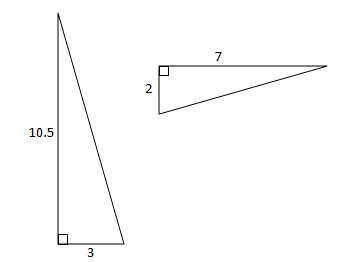No

Yes - scale factor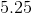Yes - scale factor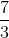Yes - scale factorYes - scale factor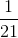Yes - scale factorExplanation:

For both triangles, we are given the "legs." Based on their relative lenghts, we can see that 2 corresponds with 3, and 7 corresponds with 10.5. First we need to make sure that these two triangles are similar. We can do this by comparing the ratios of corresponding sides: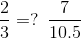There are a couple of ways to go from here. One would be to cross-multiply: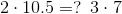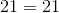the ratios are equal, so the triangles are similar, and the scale factor is.

### Example Question #6 : Similar Triangles

Are these triangles similar? If so, state the scale factor.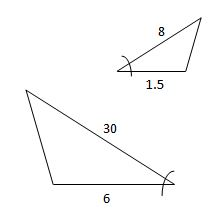No, they are not similar

Yes - scale factorYes - scale factorNot enough information

Yes - scale factorNo, they are not similar

Explanation:

Based on their positions relative to the congruent angles, and their relative lengths, we can see that 1.5 corresponds to 6, and 8 corresponds to 30. If the ratios of corresponding sides are equal, then the triangles are congruent: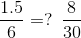We can compare these in a couple different ways. One would be to cross-multiply: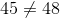These triangles are not similar.

### All New SAT Math - Calculator Resources